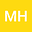A study the effect of the loss functions on E-Bayesian estimation and E-MSE for reliability parameters
•• Ming Han
Ming Han
Ningbo University of Technology

Corresponding Author:hanming618@21cn.com

Author Profile## Abstract

In order to measure the estimated error, the definition of E-MSE(expected mean squared error) is introduced based on the definition of E-Bayesian estimation. Moreover, under different loss functions(include: squared error loss, K-loss function, precautionary loss and entropy loss), the formulas of E-Bayesian estimation and E-MSE for reliability parameters of exponential distribution are given respectively. Monte Carlo simulations are performed to compare the performances of the proposed methods of estimation and a real data set have been analysed for illustrative purposes(also using OpenBUGS), results are compared on the basis of E-MSE. When considering evaluating the E-Bayesian estimations under different loss functions, this paper proposed the E-MSE as evaluation standard.